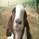1274 views
1274
This indicator is a modification of Golgistain's Hi/Lo Pivot indicator. I added a shorter length 2 to get early entries on short positions.

The way it works is you choose a timeframe you would like to trade and then set the indicator length to match the candle price action patterns. Default is M5 chart with 4/2 for the indicators.

Rules: Take the trade when price breaks out the top or bottom of the line.
Exit: Take what you can get and give nothing back.

Good luck - CharlieMax :0)
// Original work done by Golgistain
// Modified by Charlie Max for price action scalping

study(title="HB H/L", shorttitle="ScratchiCloud", overlay=true)
len = input(4, minval=1, title="Length")
len2 = input(2, minval=1,title="Length")
//The length defines how many periods a high or low must hold to be a "relevant pivot"

h = highest(len)
//The highest high over the length
h1 = dev(h, len) ? na : h
//h1 is a pivot of h if it holds for the full length
hpivot = fixnan(h1)
//creates a series which is equal to the last pivot

l = lowest(len2)
l1 = dev(l, len2) ? na : l
lpivot = fixnan(l1)
//repeated for lows

plot(hpivot, color=blue, linewidth=2)
plot(lpivot, color=purple, linewidth=2)
//plot(hpivot, color=blue, linewidth=2, offset= -len+1)
//plot(lpivot, color=purple, linewidth=2, offset= -len2+1)
//plot(h1, color=black, style=circles, linewidth=4, offset= -len+1)
//plot(l1, color=black, style=circles, linewidth=4, offset= -len+1)Thanks for this code...did you put the GREY rectangle areas in manually or is this part of the code? (automatic draw in). I'm going to work with this in the YM and CL futures.